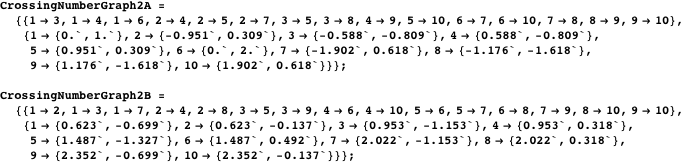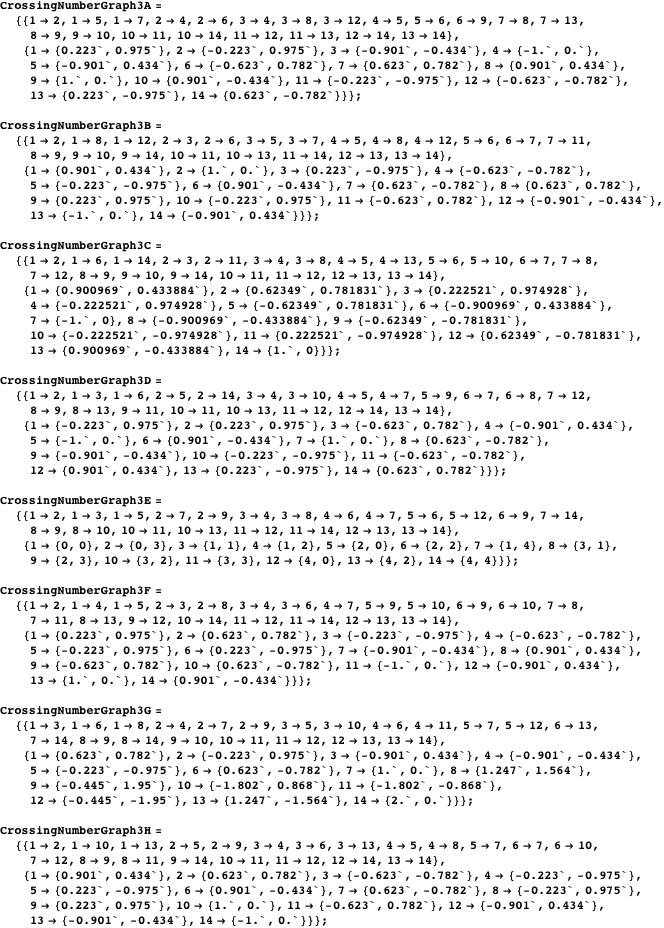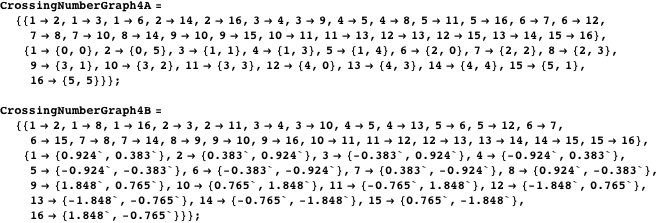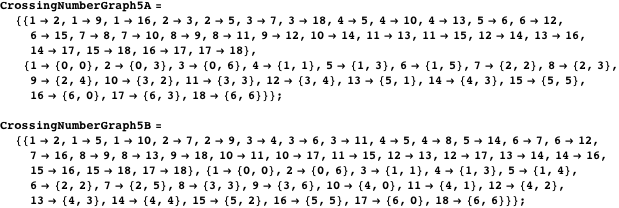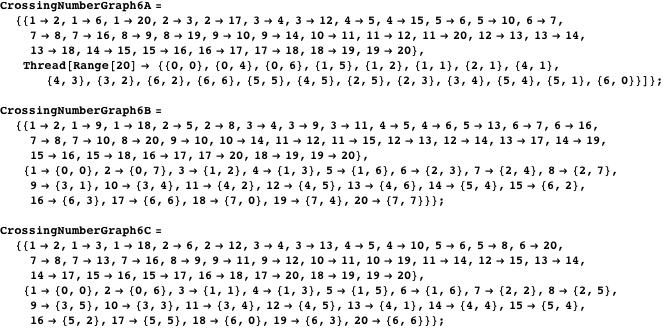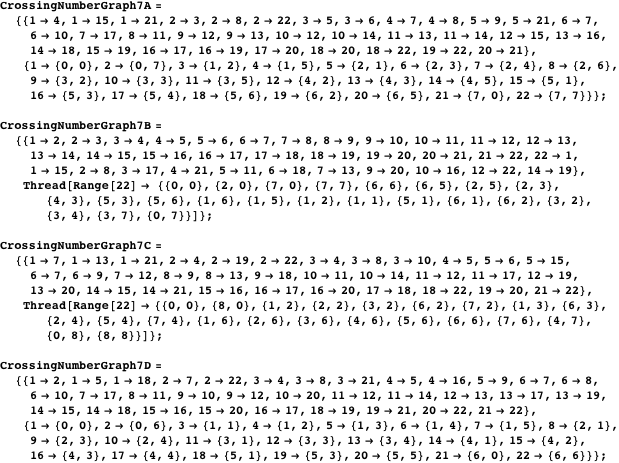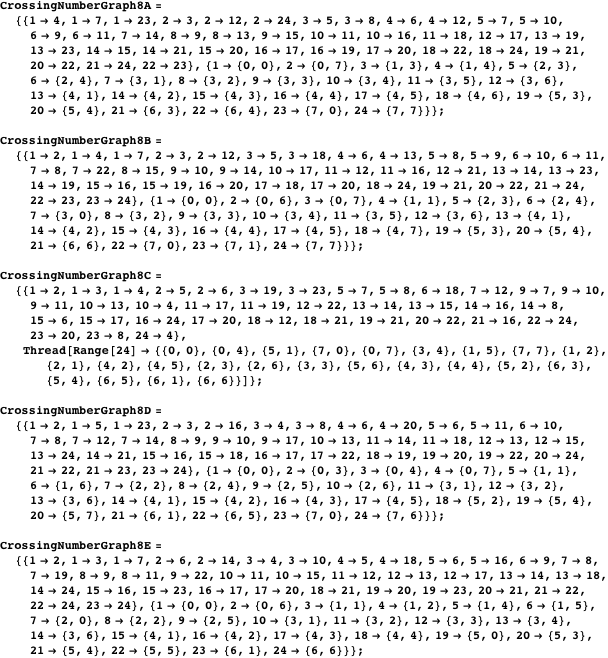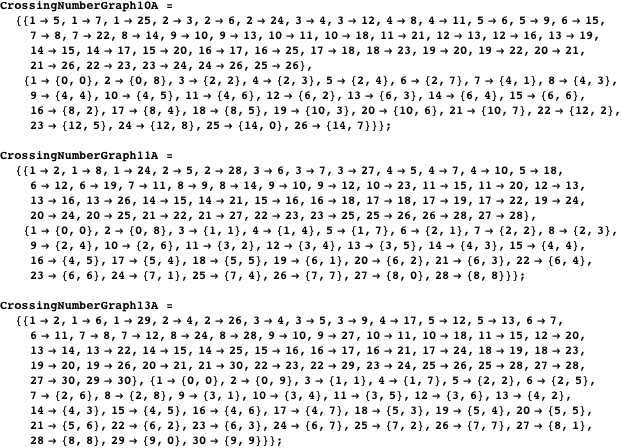Ed Pegg, Geoffrey Exoo

## Crossing Number GraphsNBCDFPDF

### B E Y O N D   S U D O K U

Sudoku is just one of hundreds of great puzzle types. This column presents obscure logic puzzles of various sorts and challenges the readers to solve the puzzles in two ways: by hand and with Mathematica. For the latter, solvers are invited to send their code to edp@wolfram.com. The person submitting the most elegant solution will receive a prize.

### The Brick Factory

In 1940, the Hungarian mathematician Paul Turán was sent to a forced labor camp by the Nazis. Though every part of his life was brutally controlled, he still managed to do serious mathematics under the most extreme conditions. While forced to collect wire from former neighborhoods, he would be thinking about mathematics. When he found scraps of paper, he wrote down his theorems and conjectures. Some of these scraps were smuggled to Paul Erdős. One problem he developed is now called the brick factory problem.

We worked near Budapest, in a brick factory. There were some kilns where the bricks were made and some open storage yards where the bricks were stored. All the kilns were connected to all the storage yards. The bricks were carried on small wheeled trucks to the storage yards. All we had to do was to put the bricks on the trucks at the kilns, push the trucks to the storage yards, and unload them there. We had a reasonable piece rate for the trucks, and the work itself was not difficult; the trouble was at the crossings. The trucks generally jumped the rails there, and the bricks fell out from them, in short this caused a lot of trouble and loss of time which was precious to all of us. We were all sweating and cursing at such occasions, I too; but nolens volens the idea occurred to me that this loss of time could have been minimized if the number of crossings of the rails had been minimized. But what is the minimum number of crossings? I realized after several days that the actual situation could have been improved, but the exact solution of the general problem with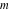kilns andstorage yards seemed to be very difficult. The problem occurred to me again at my first visit to Poland where I met Zarankiewicz .

### Complete Graphs

Turán built the field of extremal graph theory while a prisoner. Many good puzzles come from graphs, such as the familiar problem of connecting water, gas, and electricity to three houses without the lines crossing. This would be equivalent to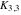or 3 kilns, 3 storage yards. Without some form of cheating, the problem is impossible, since one crossing is required. (Try proving the impossibility with Euler’s formula: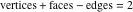). Consider the brick factory problem with 4 kilns at the top, and 3 storage yards at the bottom, or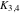. What is the minimal number of crossings? If you are looking at the notebook version of this column in Mathematica 6 or later, you can drag the vertices—first double-click a vertex twice.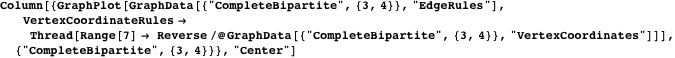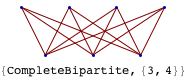For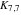, in 1970 Kleitman proved the minimal number of crossings was 77, 79, or 81 . He ended his paper with “Which?”—a question not solved until 1993, when Woodall showed the answer was 81 .

For many years, the number of crossings for the complete graph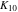was known to be either 61 or 62, with a proof of 62 finally arriving in 2000 . Below are pictures of the graph in two forms, one symmetric, the other with minimal crossings. Due to the tediousness of counting that many crossings by hand, and the 40-plus years required to solve it, I do not consider this a friendly puzzle for casual solving. The Rectilinear Crossing Number (RCN) project, maintained by Oswin Aichholzer, has solved all complete graphs up to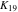, with 1318 crossings . The calculation and bounding of crossing numbers remains active to this day [6, 7].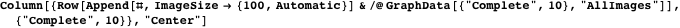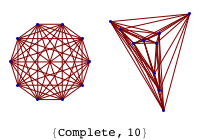### GraphData

GraphData is an extensive built-in data paclet included with Mathematica 7 . It contains more than 3000 graphs, in excess of 200 graph properties, and one or more attractive embeddings for most of its constituent graphs. GraphData has been painstakingly compiled by Eric Weisstein and myself making use of extensive Mathematica computations, programming, hand-drawings, careful mining of the graph theoretic literature, and feedback from a number of prominent graph theorists. (If you have any additions to suggest for GraphData, please feel free to email them to eww@wolfram.com.) For example, here are a few 30-vertex graphs from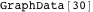.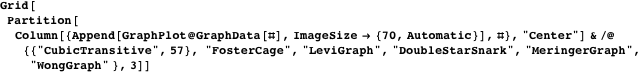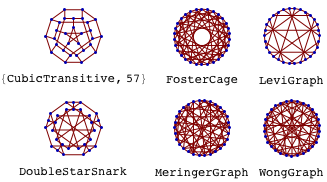### Crossing Number Graphs

A graph with no crossings is a planar graph. Here is a Wolfram Demonstration (“Planarity”) for solving planar graph embedding puzzles [9, 10]. You can drag vertices.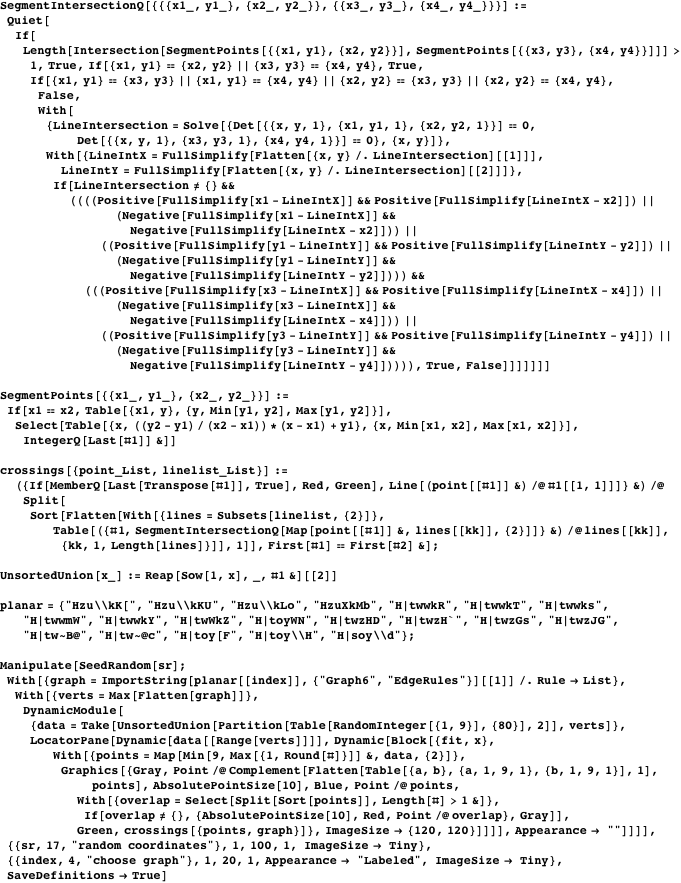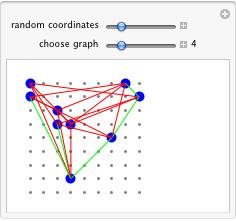One piece of research I did, with substantial help from Geoffrey Exoo , was to catalog the smallest cubic graphs that require a certain number of crossings. I named these objects “crossing number graphs”, or CNGs in this column. We have already met CNG 1, sincerequires one crossing. With two crossings, there are two minimal cubic graphs, one the famous Petersen graph.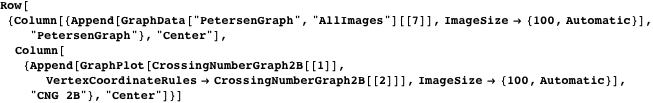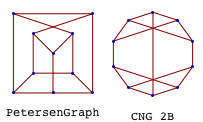With the 3-crossing graphs, there are eight graphs to choose from, with two of them being famous. CNG 3E has been shown in a 3-crossing form; the rest are given as embedding puzzles.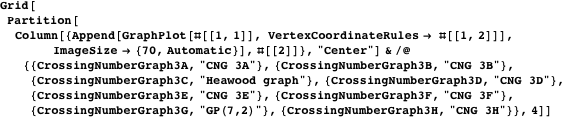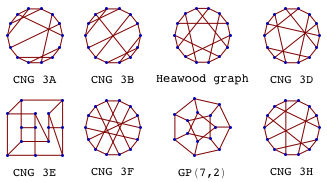In 4-crossing graphs, both are famous. The crossed-prism graph has been solved for you. The 4-crossing version of the Möbius-Kantor graph can probably be improved. This comment applies to almost all embeddings in this column.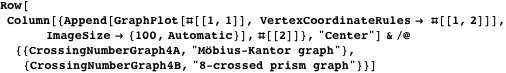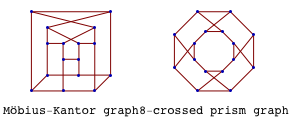With 5-crossing cubic graphs, one is famous. So far, all the crossing number graphs included a famous graph, and I thought I was seeing a trend. Both of these are difficult embedding puzzles.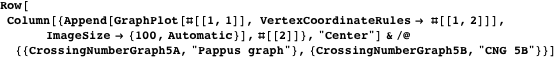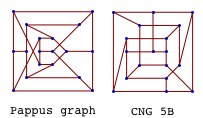At 6 crossings, all three graphs were incidence graphs for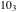configurations. Configuration puzzle: arrange 10 points to make 10 lines of three points, with three lines through each point. There are 10 such configurations . Again, one famous graph.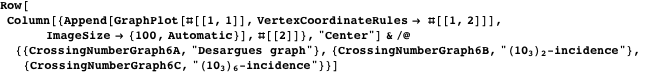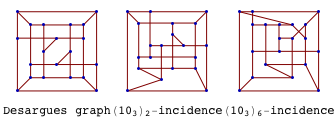The trend of crossing number graphs being famous was shattered with the 7-crossing graphs. There are four such graphs, and none of them seems to be famous.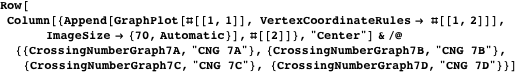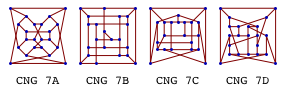The five 8-crossing graphs contain the McGee graph and Foster 24A.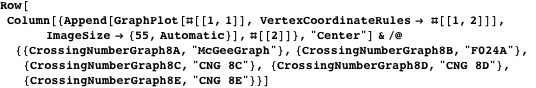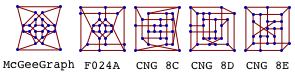With 26 vertices and beyond, the authors make conjectures. With 26, 28, and 30 vertices, the conjectured maximal crossings are 10, 11, and 13. Note that 9 crossings would not be extremal. The authors welcome expansion of these results. A related existence question, still unsolved, involves the smallest cubic graph such that the crossing number is less than the rectilinear crossing number.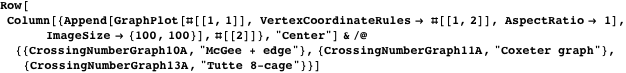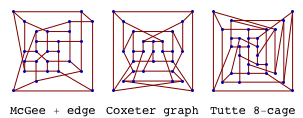No other crossing number graphs are known at this time. If you find any nice embeddings for any of these graphs, please send them to edp@wolfram.com. The crossing number graphs were found by co-author Geoffrey Exoo, using his program gnubar (nu,, represents a crossing number; a bar suggests rectilinearity). The many cubic graphs he tested were obtained from Brendan McKay’s nauty program . A brief description of the gnubar analysis of 7-crossing graphs is as follows:

1. Set as the target the highest crossing number possible. So far, adding two vertices increases the maximum crossing number by one.
2. Run a fast drawing routine that looks for reasonably good drawings for each graph. An embedding with fewer crossings than the target number eliminates that graph. For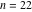, out of 1435720 graphs, 50 graphs remained.
3. A different randomized graph embedding routine found drawings with six or fewer crossings for all but the four graphs.
4. Prove that there are no better drawings for these four graphs. This step takes the most time—roughly 150 hours per graph.

### Solution for Number Link (Issue 10:3)

#### Recap of Number Link Rules

As mentioned in Volume 10, Issue 3, a Number Link puzzle follows four basic rules:

1. A continuous line must connect identical numbers.
2. Lines must go through the center of a cell horizontally or vertically and never go through the same cell twice.
3. Lines cannot cross, branch off, or go through other numbered cells.
4. Every unnumbered square must contain part of a path.

The only person sending in a solving method was Don Knuth.

“Has anybody to your knowledge pointed out that there’s an easy way to “reduce” Number Link to finding Hamiltonian paths? Namely, suppose there are four links, with the digit 1 in cells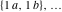, and digit 4 in cells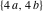. Extend the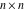grid graph by adding four new vertices , , , , and eight new edges 1b——2a, 2b——3a, 3b——4a, 4b——1a. A solution to the original puzzle is equivalent to a Hamiltonian path in this new graph.”

#### Don Knuth’s Method

First, for one of the puzzles, five extra points are added to make the base graph.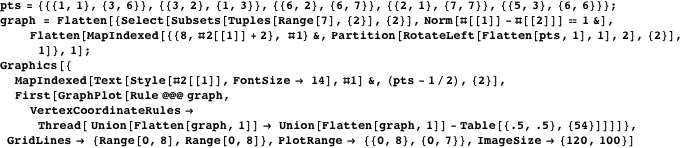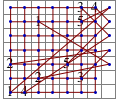After that, all Hamiltonian cycles for the graph are found—66648 different cycles in total. The desired graph has the defining point set in order.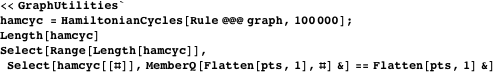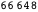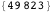From that, the solution immediately follows.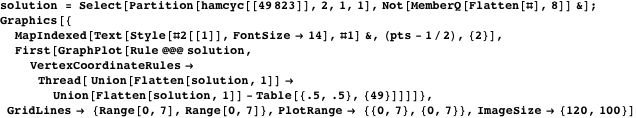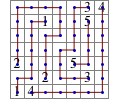For solving, Don Knuth has received the Nikoli Puzzle Cyclopedia.

### References

  P. Turán, “A Note of Welcome,” Journal of Graph Theory, 1(1), 1977 pp. 7-9.  D. J. Kleitman, “The Crossing Number of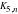,” Journal of Combinatorial Theory, 9, 1970 pp. 315-323.  D. R. Woodall, “Cyclic-Order Graphs and Zarankiewicz’s Crossing-Number Conjecture,” Journal of Graph Theory, 17(6), 1993 pp. 657-691. doi.10.1002/jgt.3190170602.  A. Brodsky, S. Durocher, and E. Gethner. “The Rectilinear Crossing Number of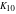Is 62.” (Sep 22, 2000) arxiv.org/abs/cs/0009023.  O. Aichholzer. “On the Rectilinear Crossing Number.” (2006) www.ist.tugraz.at/staff/aichholzer/research/rp/triangulations/crossing.  E. de Klerk, J. Maharry, D. V. Pasechnik, R. B. Richter, and G. Salazar. “Improved Bounds for the Crossing Numbers of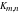and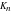.” (Apr 6, 2004) arxiv.org/abs/math/0404142.  “GraphData” from the Wolfram Mathematica Documentation Center. reference.wolfram.com/mathematica/ref/GraphData.html.  J. Pach, J. Spencer, and G. Tóth, “New Bounds on Crossing Numbers,” in Proceedings of the Fifteenth Annual Symposium on Computational Geometry (SCG’99), Miami Beach, FL (V. Milenkovic, ed.), New York: ACM Press, 1999 pp. 124-133. citeseer.ist.psu.edu/520124.  E. Pegg Jr. “Planarity” from The Wolfram Demonstrations Project. demonstrations.wolfram.com/Planarity.  E. W. Weisstein. “Planar Graph” from MathWorld—A Wolfram Web Resource. mathworld.wolfram.com/PlanarGraph.  G. Exoo. “Rectilinear Drawings of Famous Graphs.” (2006) ginger.indstate.edu/ge/Graphs/RECTILINEAR.  B. Grunbaum, “Lectures on Configurations,” in Proceedings of the International Configurations 2004 Workshop, villa Plemelj, Bled, 2004.  B. D. McKay. “The Nauty Page.” (2008) cs.anu.edu.au/~bdm/nauty. E. Pegg Jr, “Crossing Number Graphs,” The Mathematica Journal, 2011. dx.doi.org/doi:10.3888/tmj.11.2-2.

Ed Pegg Jr
Scientific Information Editor
Wolfram Research, Inc.
edp@wolfram.com

Geoffrey Exoo
Professor of Mathematics and Computer Science
Indiana State University
Terre Haute, IN 47809
gexoo@indstate.edu

##### Initialization